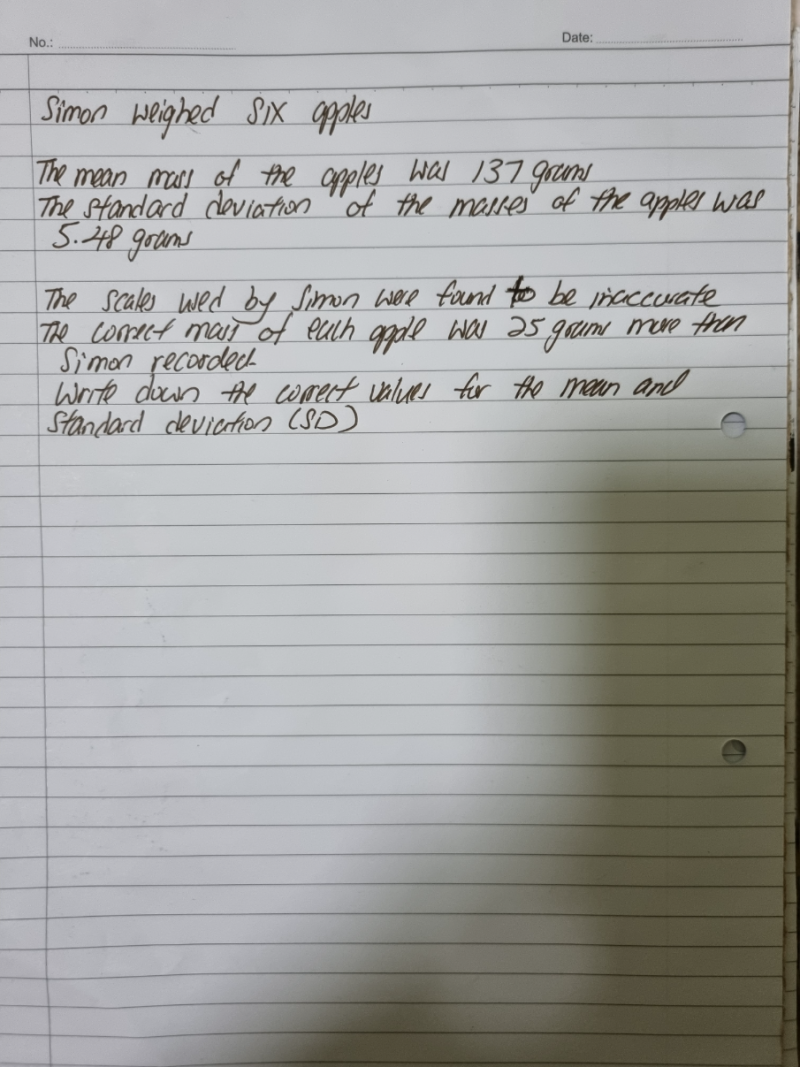# QuestionThank you

Mean is a measure of average that divides up the total equally among the number of data points. If the mass of each apple increases by 25 grams, there is an equal number of additional “25 grams”s to be distributed to each of the six apples. Hence, the mean simply increases by 25 grams.

mean = 137 g +25 g = 162 g

Standard deviation is the measure of how far the data set is spread out or away from the mean. The mean increases by 25 grams but so does every single data point (i.e. each apple). This means there is no difference in the standard deviation if every apple increases by the same amount since the mean will simply increase by that amount as well.

standard deviation = 5.48 g

0 Replies 2 Likes ✔Accepted Answer

This questions tests the conceptual understanding of definition of mean and sd.

An alternative solution is to calculate the new mean if one cannot explain why.

Original mean = 137     Apples = 6       Total = 137 x 6 = 822

Increase in each apple = 25   =>  Total increase = 25 x 6 = 150

New total = 822 + 150 = 972       Hence New mean = 972/6 = 162 g

Since the increase in mass for each apple is a constant, this implies the difference in mass between each apple is the same.  By definition, the standard deviation is the spread from the mean, hence it remains the same.

Standard deviation has no change = 5.48g

0 Replies 2 Likes